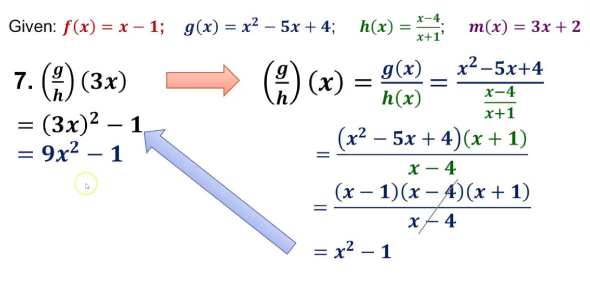# Question On General Mathematics

15 Questions | Total Attempts: 150SettingsRelated Topics
• 1.
What is the sum of all nos. between 1 and 1000 that are divisible by 5 but not by 2?
• A.

30000

• B.

50000

• C.

55000

• D.

40000

• 2.
If a die is thrown once, what will be the probability of getting a prime number?
• A.

10-Sep

• B.

5-Mar

• C.

2-Jan

• D.

3-Feb

• 3.
If tan A = cot B, what will be the value of A + B?
• A.

45 degrees

• B.

• C.

• D.

• 4.
The tangents drawn at the ends of a diameter of a circle are _____.
• A.

• B.

• C.

Perpendicular

• D.

• 5.
Which of these are main components of nichrome?
• A.

Zinc-Iron

• B.

Nickel-Tin

• C.

Nickel-Chromium

• D.

Iron-Nickel

• 6.
If a/3 = b/4 = c/7, then what will be the value of (a+b+c) ≈ c?
• A.

12

• B.

6

• C.

2

• D.

8

• 7.
• A.

8

• B.

10

• C.

6

• D.

12

• 8.
A line of 7.5cm was wrongly measured as 6.9cm. What is the error %?
• A.

12%

• B.

10%

• C.

16%

• D.

8%

• 9.
If 8700 ≈ x = 300 and 4590 ≈ y = 170, then what is x^2 - y^2?
• A.

112

• B.

116

• C.

158

• D.

100

• 10.
What number, when added to 25 and 37, comes to 5:6?
• A.

35

• B.

25

• C.

42

• D.

40

• 11.
What will be the value of 1 degree Celsius temperature in Fahrenheit scale?
• A.

33.8 degrees

• B.

60 degrees

• C.

72 degrees

• D.

45 degrees

• 12.
Boart is a form of which of these?
• A.

• B.

Coal

• C.

• D.

• 13.
Aqueous solution of cardon-di-oxide turns blue litmus to what color?
• A.

Red

• B.

White

• C.

Pink

• D.

Green

• 14.
If a-b = 4 and ab = 21, what is the value of a+b?
• A.

10

• B.

18

• C.

12

• D.

22

• 15.
When a photon transfers momentum to an electron, it's wavelength ____.
• A.

Increases

• B.

Doubles

• C.

Decreases

• D.

Remains same